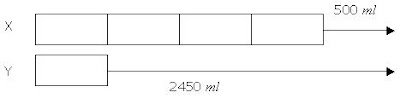This blog is managed by Song Hock Chye, author of Improve Your Thinking Skills in Maths (P1-P3 series), which is published and distributed by EPH.

## Wednesday, January 09, 2008

### Ai Tong School P5 Math CA1 2006 (Q45)

Jug X contains 4 times as much syrup as Jug Y. Sam adds 500ml of syrup to Jug X and 2 450 ml to Jug Y. Now the two jugs have the same amount of syrup. What is the total amount of syrup in the jugs now?

Solution3 units ----- 2450 – 500 = 1950

1 unit -----1950 divided by 3 = 650

Total ----- 5 units + 500 + 2450

= (5 x 650) + 500 + 2450

= 3250 + 500 + 2450

= 6200

Answer: The total amount of syrup now is 6 200 ml